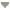出版日期: 2017-01-25点击次数：下载次数：DOI: 10.11834/jrs.201760232017 | Volumn21 | Number 1 上一篇|

 收稿日期: 2016-01-28; 修改日期: 2016-06-17; 优先数字出版日期: 2017-01-25 基金项目: 国家自然科学基金（编号：41101407，61301278）；湖北省自然科学基金（编号：2014CFB377，2010CDZ005）；华中师范大学中央高校基本科研项目（编号：CCNU15A02001）；武汉市晨光计划（编号：2016070204010137）；华中师范大学中央高校基本科研业务费项目（编号：CCNU16JCZX09） 第一作者简介: 李畅(1982-), 男, 副教授, 研究方向为地理信息科学、数字摄影测量与遥感的理论、技术和地学应用研究。E-mail:lcshaka@126.com; lichang@mail.ccnu.edu.cn 中图分类号: N93 文献标识码: A 文章编号: 1007-4619(2017)01-0074-10

# 关键词

Uncertainty estimation, control, and analysis of fractal dimension for urban traffic networkLI Chang , LI Gui'e , ZHU Yujia
Key Laboratory for Geographical Process Analysis and Simulation, Hubei Province, College of City and Environmental Science, Central China Normal University, Wuhan 430079, China

# Abstract

Finding the ideal fractal of the objective world based on pure mathematics is difficult, but the statistical significance of random fractal is an objective existence such that fractal and fractal dimension has some uncertainty. The length-radius dimension model is a fractal dimension method used to describe the complex and uncertain phenomenon of urban traffic network. However, the uncertainty of the model is frequently neglected, and related research are rarely reported. Therefore, theories and methods of uncertainty for fractal dimension should be developed and improved urgently. Aiming at the uncertainty existing in the measuring process of length-radius dimension model, we first systematically conduct research on the analysis, quantitative estimation, and quality control of fractal dimension for the urban traffic network.

The uncertainty estimation and analysis of this model are conducted from the aspects of data source, vector processing, measuring center, scale selection, and fractal dimension estimation. In particular, the uncertainty measurement interval of fractal dimension (i.e., the corresponding regression coefficient) under a certain confidence level is first provided quantitatively. Then, based on the theory of error propagation, we describe the propagation and accumulation of errors. Meanwhile, a method of quality control is proposed using least median of squares (LMedS) to remove the gross error (i.e., outliers) and to determine the scale less range simultaneously.

In this study, the experimental data were selected from the traffic network distribution map of Lhasa City in 2011, which is the map of the Lhasa road network with a scale of 1:370000 published by the China Cartographic Publishing House. The main road includes national, provincial, county, and township roads. The Lhasa City traffic network distribution map was acquired by registration and vectorization using ArcGIS. Experimental results show that vectorization of the traffic network and selection of the measuring center and scale cause uncertainty in the fractal dimension. The road is vectorized under different scale environments, e.g., 1:1000, 1:10000, cdots, 1:500000. Thus, the uncertainty for roads can be obviously observed. Transportation hub and geometric centers are employed to verify the uncertainty of fractal dimension. The uncertainty of the length-radius dimension model is controlled at a certain level of probability, and the uncertainty of this model is measured by calculating the confidence interval for the first time. To be exact, the confidence interval of the fractal dimension is (1.633, 1.707) under the confidence level of 95%. Furthermore, the corresponding table of the original data and data processed by LMedS proves that the proposed method is reliable, that is, the coefficient of determination R2 is improved from 0.9931 to 0.9989.

From the description of the uncertainty of fractal geometry and fractal dimension, this study systematically reveals the uncertain essence of the model. The proposed methods of uncertainty, quality control, and analysis are not only applicable to the length-radius dimension model but also to the branch dimension that is similar to the calculation method. The model can also provide references for the statistical significance of all random fractals in nature. The proposed model not only further enriches fractal dimension theory and establishes the theoretical foundation of its quality control but also provides reliable scientific basis for the geoscience applications of fractal dimension for the urban traffic network.

# Key words

traffic network , length-radius dimension model , uncertainty , LMedS , quality control , error propagation

# 2 分形维数的不确定性分析原理与质量控制

 ${L^{1/1}} \propto {S^{1/2}} \propto {V^{1/3}}$ (1)

 ${L^{1/1}} \propto {S^{1/2}} \propto {V^{1/3}} \propto {M^{1/D}}$ (2)

 $L{\left( S \right)^{1/D}} \propto {S^{1/2}}$ (3)

 $L\left( r \right) = {L_1}{r^D}$ (4)

## 2.1 分形维数的不确定性分析原理

(1) 数据源的不确定性。对于研究学者而言，城市交通道路网的基础数据源主要来自于对纸质或电子地图的矢量化并同时辅助其他工具对数据进行完善。从数据获取源来看，影响数据质量的因素主要包括地图测量误差、绘制误差，图纸变形误差(柳宗伟 等，2002)，地图要素本身的密度和复杂程度以及地图综合(王桥 等，1998; 艾廷华 等，2007; Li，2007)过程中对于地物的取舍程度等。

(2) 数据转换的不确定性。从城市交通道路矢量数据处理方式来看，数字化以及数字化操作人员的熟练程度及操作方式、数据格式转换等同样存在不确定性。对于同一区域，不同比例尺的地图对分维数会产生影响(陈杰和马素媛，2012)，即使是同一比例尺的地图，在不同比例的矢量化环境下，其结果也不相同，从而导致不确定性。故，矢量化的结果，例如：点、线、面皆存在不确定性，其中点的不确定性可以由误差椭圆来描述；线的不确定性可以由G-带误差模型进行概括；面的不确定性则可通过讨论多边形组成顶点的不确定性和基于多边形边界的误差带两种方法对其不确定性进行度量(Shi，2009)。

(3) 测算中心和测算尺度的不确定性。对交通道路网的长度-半径维数进行测算时需要选择测算中心和测算尺度。从当前学者的研究工作来看，测算中心或为城市交通网络的枢纽或为城市的几何中心亦或是重心，不同的选择所获得结果也不尽相同，故在中心选择上存在认知的不确定性。即使同是选择城市道路网的交通枢纽中心，也会因研究者不同，而有差别，即相应测算中心都存在一个点位误差(测算点与真实点)，可用点位误差椭圆(Shi，2009)来衡量。对于测算尺度的选取，就目前而言，没有固定的标准，多数学者都是结合研究区域的特征选取合适的尺度进行测算，这也为测算区域交通网络的分维值增加了多样性。

(4) 分形维数估计的不确定性度量。对于分形维数模型，有些不确定性可以通过对数据重新定位、浏览、查错等进行降低，而有些则是无法避免且随处理过程进行误差传播累积。对于此类不确定性可通过计算分维数的置信区间进行定量估计。

 $\ln L\left( r \right) = \ln {L_1} + D\ln r$ (5)

 ${y_i} = \hat b{x_i} + \hat a + {\varepsilon _i}$ (6)

 $\left\{ \begin{array}{l} \hat b = \displaystyle \frac{{{S_{xy}}}}{{{S_{xx}}}}\\ \hat a = {\displaystyle{\frac{1}{n}}}\sum\limits_{i = 1}^n {{y_i}} - \left( {{\displaystyle{\frac{1}{n}}}\sum\limits_{i = 1}^n {{x_i}} } \right)\hat b \end{array} \right.$ (8)

 $\mathop {\min }\limits_{\hat \beta } \left[ {\mathop {{\rm{med}}}\limits_i \left( {r_i^2} \right)} \right]$ (17)

 ${{\hat \sigma }_S} = 1.4826\left( {1 + \frac{5}{{\left( {n - p} \right)}}} \right)\sqrt {{M_J}}$ (18)

 ${W_i} = \left\{ \begin{array}{l} 1\;\;\;\;r_i^2 \leqslant {\left( {2.5{{\hat \sigma }_S}} \right)^2}\\ 0\;\;\;\; \text{其他} \end{array} \right.$ (19)

# 3 算例与分析

## 3.1 数据简介

Fig. 1 The traffic network distribution of Lhasa in 2011

## 3.2 实验结果与分析

(1) 矢量化过程中的不确定性。在获取基础数据时，有些客观因素导致的不确定性是无法避免的，但有些却是可以人为控制的。在对拉萨市道路矢量化过程中发现：对于同一交通网络，不同比例尺的矢量化环境中得到的道路长度差别很大。本文以国道318在达孜县的一段进行矢量化为例，选择同样的起始点和矢量化边界(道路的南侧边界)，在不同比例尺的矢量化环境下对其进行了矢量化，其结果如图 2所示。

Fig. 2 The road length and partial enlarged details under different scale vectorization environment

(2) 测算中心不同所引起的分维数的差异。在对拉萨市交通网络分维数进行测算时，不同测算中心和测算尺度测得的分维数存在一定差异。结合拉萨市交通网络的分布特征，分别以拉萨市(城关区)交通枢纽中心和拉萨市的几何中心为测算中心，对其分维数进行测定。在测算尺度选择方面，以交通枢纽为测算中心的数据以$r$=10 km进行处理，以几何中心为测算中心的数据分别以$r$=10 km、$r$=12.5 km、$r$=15 km进行处理，获得了相应的点列(ln$r$，ln$L$($r$))，并对其进行拟合，其结果如图 3所示。

Fig. 3 The ln-ln plots on the lengths of traffic network against radial distances of Lhasa under different measuring center and measuring scale

(3) 基于LMedS的数据质量控制。在实验数据的获取过程中，受各种条件限制，再加上数据本身也存在很大的不确定性，故数据有可能存在异常值，所以在对拉萨市交通网络的数据进行拟合之前，本文首先对拟合数据做了基于LMedS的数据质量控制。借助MATLAB编写的程序对以交通枢纽为中心的数据进行了处理。数据处理结果如表 1表 2所示。

Table 1 The original data

 标号 1 2 3 4 5 6 7 8 9 10 11 12 13 14 15 16 ln$r$ 2.303 2.996 3.401 3.689 3.912 4.094 4.248 4.382 4.5 4.605 4.7 4.787 4.868 4.942 5.011 5.075 ln$L$($r$) 5.092 6.16 6.882 7.376 7.734 8.012 8.272 8.546 8.786 8.958 9.093 9.187 9.26 9.287 9.308 9.325

Table 2 Data processed by LMedS

 标号 1 2 3 4 5 6 7 8 9 10 11 12 13 ln$r$ 2.303 2.996 3.401 3.689 3.912 4.094 4.248 4.382 4.5 4.605 4.7 4.787 4.868 ln$L$($r$) 5.092 6.16 6.882 7.376 7.734 8.012 8.272 8.546 8.786 8.958 9.093 9.187 9.26

Fig. 4 The ln-ln plot on the lengths of traffic network against radial distances of Lhasabased on LMedS

(4) 分维数的不确定性度量。对城市交通网络分布图进行了矢量化、选取测算中心、建立缓冲区、数据异常值去除等基本数据处理之后，按照长度-半径维数模型对其分维数进行测算。本文以拉萨市交通枢纽中心为测算中心，并根据式(14)对其分维数的不确定性进行了度量，根据式(15)对回归直线的置信域进行了求解和绘制出图，则在满足置信水平95%的情况下分形维数的置信区间为(1.633，1.707)，其对应的分布情况如图 5所示。

Fig. 5 The confidence interval of regression function of the length-radius dimension model

# 4 结论

(1) 从不确定性来源来看，主要包括道路网的选择和路网的矢量化过程、测算中心和测算尺度的选取、实验数据的不确定性、模型本身具有的不确定性等方面。

(2) 对分维数的测算过程是一个完整连续的过程，依据误差传播累积理论，可以首先基于LMedS去除异常值数据从而提高可靠性，继而通过计算分维数满足一定置信水平的置信区间对其不确定性进行度量，用于辅助分析城市交通网络的分形分维情况。